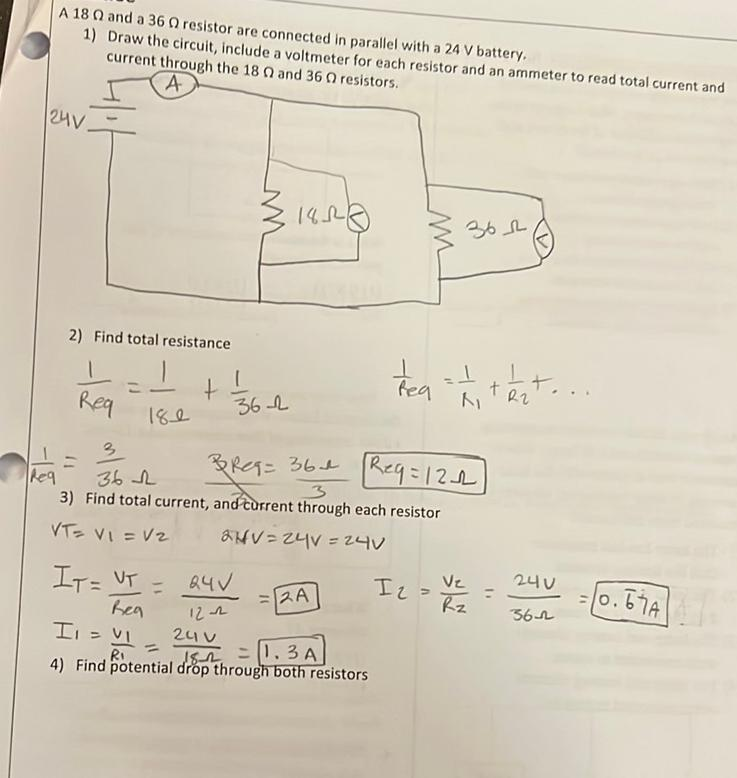Question:

# A 18 0 and a 36 resistor are connected in parallel with a 24 V battery 1) Draw the circuit, include a voltmeter for each resistoA 18 0 and a 36 resistor are connected in parallel with a 24 V battery 1) Draw the circuit, include a voltmeter for each resistor and an ammeter to read total current and current through the 18 and 36 resistors. A 124v 1950 W 362 2) Find total resistance treat test, + + 362 . Req R2 180 3 362 1 BReq=360 Req=122 Req 3) Find total current, and current through each resistor VT- Vi = V2 BHV-24V = 24V IT = VT = I 2 = RA 240 Ve Rz = 0.67A 362 24V Reg 122 uv RI 4) Find potential drop through both resistors II - VI 182 1.3A